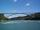# Chord - TS v2

The radius of circle k measures 87 cm. Chord GH = 22 cm. What is TS?

Result

|TS| =  0.7 cm

#### Solution:Leave us a comment of example and its solution (i.e. if it is still somewhat unclear...):Be the first to comment!#### To solve this example are needed these knowledge from mathematics:

Pythagorean theorem is the base for the right triangle calculator.

## Next similar examples:

1. Common chordTwo circles with radius 17 cm and 20 cm are intersect at two points. Its common chord is long 27 cm. What is the distance of the centers of these circles?
2. Circle chordDetermine the radius of the circle in which the chord 6 cm away from the center of the circle is 12 cm longer than the radius of the circle.
3. Circle annulusThere are 2 concentric circles in the figure. Chord of larger circle 10 cm long is tangent to the smaller circle. What are does annulus have?
4. Sphere cutsAt what distance from the center intersects sphere with radius R = 56 plane, if the cut area and area of the main sphere circle is in ratio 1/2.
5. RT and circlesSolve right triangle if the radius of inscribed circle is r=9 and radius of circumscribed circle is R=23.
6. Isosceles IVIn an isosceles triangle ABC is |AC| = |BC| = 13 and |AB| = 10. Calculate the radius of the inscribed (r) and described (R) circle.
7. BridgeThe bridge arc has a span 235 m and height 3 m. Calculate the radius of the circle arc of this bridge.
8. Circle - AGFind the coordinates of circle and its diameter if its equation is: ?
9. CenterCalculate the coordinates of the circle center: ?
10. Theorem proveWe want to prove the sentence: If the natural number n is divisible by six, then n is divisible by three. From what assumption we started?
11. Vector 7Given vector OA(12,16) and vector OB(4,1). Find vector AB and vector |A|.
12. Euclid2In right triangle ABC with right angle at C is given side a=27 and height v=12. Calculate the perimeter of the triangle.
13. ABS CNCalculate the absolute value of complex number -15-29i.Given that P = (5, 8) and Q = (6, 9), find the component form and magnitude of vector PQ.Ditch with cross section of an isosceles trapezoid with bases 2m 6m are deep 1.5m. How long is the slope of the ditch?The hypotenuse of a right triangle is 41 and the sum of legs is 49. Calculate the length of its legs.In a triangle ABC with the side BC of length 2 cm The middle point of AB. Points L and M split AC side into three equal lines. KLM is isosceles triangle with a right angle at the point K. Determine the lengths of the sides AB, AC triangle ABC.